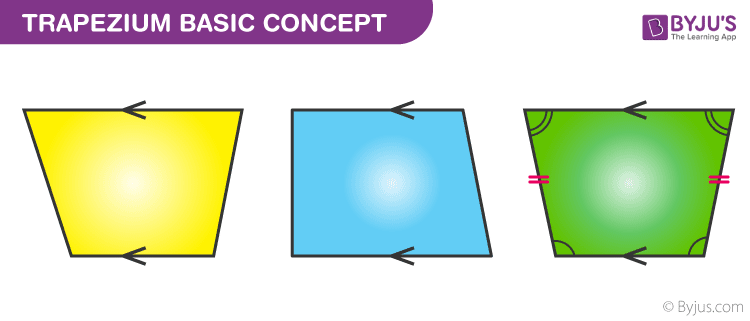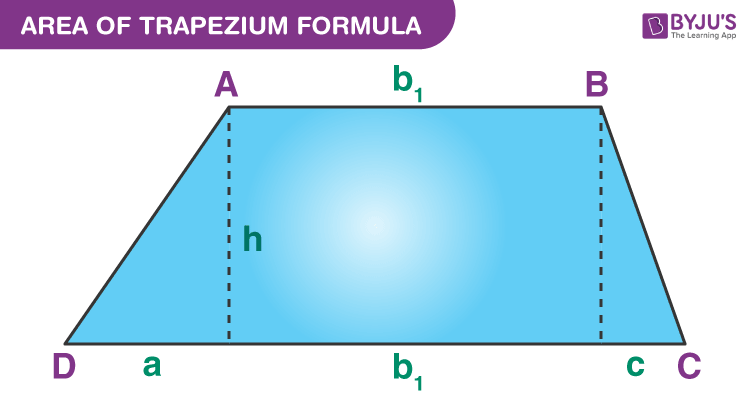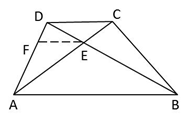# Area Of Trapezium

Area of trapezium is the region covered by a trapezium in a two-dimensional plane. It is the space enclosed in 2D geometry. A trapezium is a 2D shape that falls under the category of quadrilaterals. Similar to other geometrical shapes, it also has its own properties and formulas based on area and perimeter. Let us learn in detail.

## Trapezium Definition

A trapezium is a quadrilateral, which is defined as a shape with four sides and one set of parallel sides. Apart from trapezium, there are four more types of quadrilaterals. They are:

1. Parallelogram
2. Rectangle
3. Square
4. Rhombus

All these quadrilaterals have one common property, which is, the sum of all the angles is 360°.

### Trapezium Basic Concept

1. The pair of parallel sides are called the bases while the non-parallel sides are called the legs of the trapezoid
2. The line segment connecting the midpoints of the non-parallel sides of a trapezoid is called the mid-segment3. Check above the different types of trapezium figures, where arrow represents the parallel side of it. In all the three figures you can see, the two sides are parallel to each other, whereas the other two sides are non-parallel
4. If we draw a line segment, between the two non-parallel sides, from the mid-point of both sides,  the trapezium will be divided into two unequal parts.
5. You must have learned of isosceles triangles, where the two sides of a triangle are equal and the angle opposite the equal sides are also equal. In the same way, we have a figure, which is stated as Isosceles Trapezium, where the two non-parallel sides are equal and form equal angles at one of the bases. You can see the example of it, in the third figure given above.

## Properties of a Trapezium

Here, we are going to learn about some more properties of the trapezium, which is also called a trapezoid. A trapezium has the following properties:

1. Like other quadrilaterals, the sum of all the four angles of the trapezium is equal to 360°
2. A trapezium has two parallel sides and two non-parallel sides
3. The diagonals of regular trapezium bisect each other
4. The length of the mid-segment is equal to half the sum of the parallel bases, in a trapezium
5. Two pairs of adjacent angles of a trapezium formed between the parallel sides and one of the non-parallel side, add up to 180 degrees

## Area of a Trapezium

Trapezium area can be calculated by using the below formula:

• Area = (1/2) h (AB+CD)### Perimeter of Trapezium

The perimeter of trapezium formula is given by:

• Perimeter = Sum of all the sides =  AB + BC + CD + DA## Derivation of Area of a Trapezium

Following is the derivation for computing the area of the trapezium:

The area of a trapezoid is equal to the sum of the areas of the two triangles and the area of the rectangle.

We know that

area of trapezoid  = area of triangle 1 + area of rectangle + area of triangle 2.

That means,
A = (ah/2) + b1h + (ch/2)
A = (ah + 2b1h + ch)/2
Simplifying the equation, rearranging the terms, and factoring result to:
A = h/2[b1 + (a + b1 + c)] ….(i)
If we assume the longer base of the trapezoid be b2, then
b2 = a + b1 + c …..(ii)
Substituting (ii) in equation (i),
A = h/2(b1 + b2)

Therefore, the area of a trapezoid with bases b1, b2 and altitude h is;

A = h/2(b1+b2)

### Applications of Trapezium

The concept is a highly used concept in various physics computations and other mathematical calculations. This is the basis for obtaining the equations of motion as described in the 9th CBSE science textbook. The blend of the physics equations and mathematical calculations is very well explained to clear the level of understanding of a budding engineering mind.

For the complete understanding and concept clearance, one must go through the practice worksheets at BYJU’S to check their hands on the concept and test learning.

## Frequently Asked Questions on Area of Trapezium

### What is the formula for Area of a trapezium?

The formula to calculate the area of trapezium is:
Area = ½ x Sum of parallel sides x Distance between the parallel sides

### What are the formulas for Area and Perimeter of Trapezium?

Area of trapezium = ½ x (a+b) x h
Perimeter of trapezium = a+b+c+d
Where a, b, c and d are the length of sides of a trapezium
And h is the distance between the two parallel sides i.e., a and b.

### What are the properties of Trapezium?

A trapezium has 4 unequal sides: two parallel and two non-parallel sides
Sum of all interior angles is 360 degrees
Diagonals bisect each other

### How do you find the area of Trapezium?

The area of trapezium depends on its parallel sides and distance between the parallel sides.
If we know the length of parallel sides and the distance between them, then we can easily find the area of trapezium.

1. Nafees Malik

How the diagonals of a trapezium bisect each other?

1. balaji_byjusABCD is a trapezium.
Draw EF || BA || CD, intersecting AD in F.
FE || AB (from figure)
DE/EB = DF/FA [basic proportionality theorem] … (1)
FE || DC (from figure)
CE/EA = DF/FA [basic proportionality theorem] …(2)
From equations (1) and (2), we get
DE/EB = CE/EA
i.e., diagonals of a trapezium divide each other proportionally.
Therefore, AC and BD bisect each other
Hence, we conclude that,
Diagonals of a trapezium bisect each other

2. christan

next time pls do a video which a trapaziam and nember on it pls

3. Baibhav Singh

How to find the are of the trapizum if no hight is given

1. lavanya

With respect to the parallel sides, divide the trapezium into a rectangle and two right triangles.
Find the height of the trapezium using Pythagoras theorem and then you can find the area of trapezium.

4. AMEY SAWANT

is there any video for area of parallelogram

5. Pls give video solution to find area of trapezium using triangulation

1. lavanya

Learn to find area of the trapezium in this video.

6. Ritu dabi

Are the diagonal of trapezium angle bisector of their own angles

1. lavanya

Diagonals of an isosceles trapezium bisect the angles, if and only if, three sides are equal. (Parallel sides are unequal)